# Octacontagon

Regular octacontagon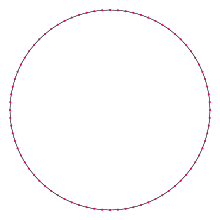A regular octacontagon
Type Regular polygon
Edges and vertices 80
Schläfli symbol {80}, t{40}, tt{20}, ttt{10}, tttt{5}
Coxeter diagramSymmetry group Dihedral (D80), order 2×80
Internal angle (degrees) 175.5°
Dual polygon Self
Properties Convex, cyclic, equilateral, isogonal, isotoxal

In geometry, an octacontagon (or ogdoëcontagon or 80-gon from Ancient Greek ὁγδοήκοντα, eighty) is an eighty-sided polygon. The sum of any octacontagon's interior angles is 14040 degrees.

## Regular octacontagon

A regular octacontagon is represented by Schläfli symbol {80} and can also be constructed as a truncated tetracontagon, t{40}, or a twice-truncated icosagon, tt{20}, or a thrice-truncated decagon, ttt{10}, or a four-fold-truncated pentagon, tttt{5}.

One interior angle in a regular octacontagon is 17512°, meaning that one exterior angle would be 412°.

The area of a regular octacontagon is (with t = edge length)The circumradius of a regular octacontagon is### Construction

Since 80 = 24 × 5, a regular octacontagon is constructible using a compass and straightedge. As a truncated tetracontagon, it can be constructed by an edge-bisection of a regular tetracontagon. This means that the trigonometric functions of π/80 can be expressed in radicals:## Symmetry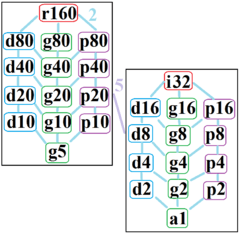The symmetries of a regular octacontagon. Light blue lines show subgroups of index 2. The left and right subgraphs are positionally related by index 5 subgroups.

The regular octacontagon has Dih80 dihedral symmetry, order 80, represented by 80 lines of reflection. Dih40 has 9 dihedral subgroups: (Dih40, Dih20, Dih10, Dih5), and (Dih16, Dih8, Dih4, and Dih2, Dih1). It also has 10 more cyclic symmetries as subgroups: (Z80, Z40, Z20, Z10, Z5), and (Z16, Z8, Z4, Z2, Z1), with Zn representing π/n radian rotational symmetry.

John Conway labels these lower symmetries with a letter and order of the symmetry follows the letter. r160 represents full symmetry and a1 labels no symmetry. He gives d (diagonal) with mirror lines through vertices, p with mirror lines through edges (perpendicular), i with mirror lines through both vertices and edges, and g for rotational symmetry.

These lower symmetries allows degrees of freedoms in defining irregular octacontagons. Only the g80 subgroup has no degrees of freedom but can seen as directed edges.

## Octacontagram

An octacontagram is an 80-sided star polygon. There are 15 regular forms given by Schläfli symbols {80/3}, {80/7}, {80/9}, {80/11}, {80/13}, {80/17}, {80/19}, {80/21}, {80/23}, {80/27}, {80/29}, {80/31}, {80/33}, {80/37}, and {80/39}, as well as 24 regular star figures with the same vertex configuration.

 Picture Interior angle Picture Interior angle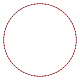{80/3}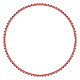{80/7}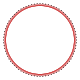{80/9}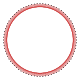{80/11}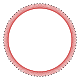{80/13}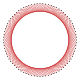{80/17}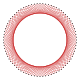{80/19}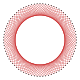{80/21} 166.5° 148.5° 139.5° 130.5° 121.5° 103.5° 94.5° 85.5°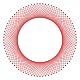{80/23}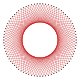{80/27}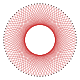{80/29}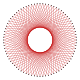{80/31}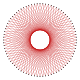{80/33}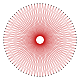{80/37}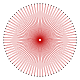{80/39} 76.5° 58.5° 49.5° 40.5° 31.5° 13.5° 4.5°
1. Greek Numbers and Numerals (Ancient and Modern) by Harry Foundalis
2. Gorini, Catherine A. (2009), The Facts on File Geometry Handbook, Infobase Publishing, p. 110, ISBN 9781438109572.
3. Constructible Polygon
4. The Symmetries of Things, Chapter 20